.form_login_top { font-family: Verdana; font-size: 7pt; color: #eee; border: 1px solid #eee; height: 17px; width: 80px; background-color: #7a3c2c; padding: 2px; } .form_login_top2 { font-family: Verdana; font-size: 7pt; color: #eee; border: 1px solid #eee; height: 17px; width: 80px; background-color: #7a3c2c; padding: 2px; }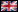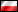Contact            Users: 108 Active, 0 Logged in - Time: 16:48Home  |  Poker Blogs  |  Poker Forum  |  Poker Articles  |  Rakeback  |  Login  |  RegisterPoker NewsLP Pokerstars Avatars:# Challenging Math problems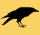FMLuser   Canada. Jun 19 2016 10:05. Posts 45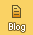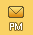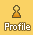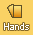I was able to solve the first 2 problems with some ease, However I am getting no where on the third and was hopping some fresh eyes from LP might be able to help

(a) Let a be a positive integer. What is gcd(a, 0)? Prove your answer is correct
using the definition of gcd.
(b) Evaluate gcd(0, 0). Is there only one possible value or many or none? Justify
(c) Prove for integers a, b > 1, that 0 < (1/a)+(1/b)-(1/lcm(a,b)) < 1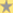0 votes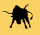Joe   Czech Republic. Jun 19 2016 11:10. Posts 5987You mean c) ? Maybe I got this wrong, but intuitively it seems quite obvious... Highest possible value is when a = b = 2: 1/2 + 1/2 - 1/2 = 1/2 < 1 For any other integers a,b > 1 the value will be smaller, so also < 1. As far as lowest possible value goes: We know that lcm(a,b) >= max(a,b) for any a,b > 1. Thus: 1/a + 1/b - 1/lcm(a,b) >= 1/a + 1/b - 1/max(a,b) = 1/min(a,b) > 0 (since a,b > 1)
 there is a light at the end of the tunnel... (but sometimes the tunnel is long and deep as hell)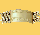Stroggoz   New Zealand. Jun 19 2016 12:04. Posts 5046is this homework for an introduction to proofs class? i agree with second half of joes proof but dont understand the first half. if a=3, b=4 then we get 1/2 when adding 1/3+1/4-1/12 =1/2, so not sure why you said a=b=2 is the highest. possible and all others are less than? You need to give a reason why a=b=2 is the highest <1, (even if its obvious) for an average math professor to give full marks i think. The way i proved that it's <1 is just to say this: since a, b >1, then 1/a +1/b is clearly <1. since a,b>1, then lcm(a,b) is positive and therefore 1/lcm(a,b) is a positive number divided by a positive number, so is therefore positive. therefore 1/a+ 1/b -1/lcm(a,b) <1 Then you can use 2nd half of Joes proof to show its >0. QED
 One of 3 non decent human beings on a site of 5 people with between 2-3 decent human beings Last edit: 19/06/2016 12:22Joe   Czech Republic. Jun 19 2016 13:14. Posts 5987Yea ok, the 1st part is not correct. So 1st part again: 1/a + 1/b <= 1 for any integers a,b > 1 (should be obvious enough to not have to prove this) And since we subtract 1/lcm(a,b), which is always > 0 (from definition of lcm), we will always get a number smaller than 1.
 there is a light at the end of the tunnel... (but sometimes the tunnel is long and deep as hell)FMLuser   Canada. Jun 19 2016 18:25. Posts 45Yeah this was the challenge question on a homework assignment, I broke the question into 2 cases where a,b is prime and where a,b is coprime and a,b > or = 4 and solved it in a similar way. Since in the prime case lcm(ab) X gcd(ab)=ab and gcd(a,b) of two primes is going to be 1 then lcm(ab)=ab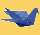Daut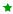United States. Jun 19 2016 18:26. Posts 8951Prove for integers a, b > 1, that 0 < (1/a)+(1/b)-(1/lcm(a,b)) < 1 For any integers a,b > 1: b <= lcm(a,b) <= ab (this is obvious, if a,b are prime then lcm(a,b) = ab, otherwise it's at least as big as each of the numbers) thus, 1/ab <= 1/lcm(a,b) <= 1/b So, 1/a + 1/b - 1/b <= 1/a + 1/b - 1/lcm(a,b) <= 1/a + 1/b - 1/ab 1/a <= 1/a + 1/b - 1/lcm(a,b) <= (a+b-1)/ab since a>1: b-1 < a(b-1) b-1 < ab-a a+b-1 < ab (a+b-1)/ab < 1 and since a>1: 1/a >0 thus, 0 < 1/a <= 1/a+1/b-1/lcm(a,b) <= (a+b-1)/ab < 1 0 < 1/a+1/b-1/lcm(a,b) < 1
 NewbSaibot: 18 TIMES THE SPEED OF LIGHT. Because FUCK YOU, Daut Last edit: 19/06/2016 18:43failsafe   United States. Jun 21 2016 09:45. Posts 1032really disagree daut because natural numbers N² N³ are enumerable... R not so. then N² ^ N³ ^ (N^n) takes us out of base ten. a,b argument is essentially bounded at log(log(26)) same with Arabic numerals (which are indian). Arabic is bounded at fingers not toes, too. So that's log(log(10) Thus the argument collapses logarithmically. you're arguing that the natural numbers you're using aren't generated by a process bounded by infinity, which is what I'm arguing but then if infinity isn't the upper bound of the process (and obviously it isn't because) ? < ?+1 < ?+2 < ... same for any non-stochastic generated natural number (positive integer) process ? < 2? < 3? < ... and so ? < ?² < ?³ < ... There's no real objection to hyperreal integers and the only obstacle to the argument is what to do about hyperreal fractions. Then even if we avoid speaking in "infinities" which is probably pretty useless as a mode of commerce (cuz infinity without some base is like a logarithm with no base) Finally we end up with the reality that there is no least common denominator to any integer process because at best the least common denominator is "an infinity". This doesn't specify which infinity and is basically useless in the same way the whole consideration is basically useless. .... You wouldn't ask me is this the least possible sequence or series in any meaningful numerical context the answer is always going to be some geometric divergence problem more like language than mathematics which is why teaching these kind of analytic questions as higher math is really idiotic. Why the hell teach something that converges to a stochastic process or some kind of error elimination approximation like numerical analysis. Pretty glad I dropped math at the logic level
 so you can show me some cardsDautUnited States. Jun 21 2016 17:23. Posts 8951On June 21 2016 08:45 failsafe wrote: really disagree daut because natural numbers N² N³ are enumerable... R not so. then N² ^ N³ ^ (N^n) takes us out of base ten. a,b argument is essentially bounded at log(log(26)) same with Arabic numerals (which are indian). Arabic is bounded at fingers not toes, too. So that's log(log(10) Thus the argument collapses logarithmically. you're arguing that the natural numbers you're using aren't generated by a process bounded by infinity, which is what I'm arguing but then if infinity isn't the upper bound of the process (and obviously it isn't because) ? < ?+1 < ?+2 < ... same for any non-stochastic generated natural number (positive integer) process ? < 2? < 3? < ... and so ? < ?² < ?³ < ... There's no real objection to hyperreal integers and the only obstacle to the argument is what to do about hyperreal fractions. Then even if we avoid speaking in "infinities" which is probably pretty useless as a mode of commerce (cuz infinity without some base is like a logarithm with no base) Finally we end up with the reality that there is no least common denominator to any integer process because at best the least common denominator is "an infinity". This doesn't specify which infinity and is basically useless in the same way the whole consideration is basically useless. .... You wouldn't ask me is this the least possible sequence or series in any meaningful numerical context the answer is always going to be some geometric divergence problem more like language than mathematics which is why teaching these kind of analytic questions as higher math is really idiotic. Why the hell teach something that converges to a stochastic process or some kind of error elimination approximation like numerical analysis. Pretty glad I dropped math at the logic level

there is no need to work with infinity, N is countably infinite. "prove for integers a,b". so to prove this, we pick random integers a,b which we assume to have values and we can proceed with proof.

 NewbSaibot: 18 TIMES THE SPEED OF LIGHT. Because FUCK YOU, Dautfailsafe   United States. Jul 03 2016 10:51. Posts 1032On June 21 2016 16:23 Daut wrote:
 On June 21 2016 08:45 failsafe wrote: really disagree daut because natural numbers N² N³ are enumerable... R not so. then N² ^ N³ ^ (N^n) takes us out of base ten. a,b argument is essentially bounded at log(log(26)) same with Arabic numerals (which are indian). Arabic is bounded at fingers not toes, too. So that's log(log(10) Thus the argument collapses logarithmically. you're arguing that the natural numbers you're using aren't generated by a process bounded by infinity, which is what I'm arguing but then if infinity isn't the upper bound of the process (and obviously it isn't because) ? < ?+1 < ?+2 < ... same for any non-stochastic generated natural number (positive integer) process ? < 2? < 3? < ... and so ? < ?² < ?³ < ... There's no real objection to hyperreal integers and the only obstacle to the argument is what to do about hyperreal fractions. Then even if we avoid speaking in "infinities" which is probably pretty useless as a mode of commerce (cuz infinity without some base is like a logarithm with no base) Finally we end up with the reality that there is no least common denominator to any integer process because at best the least common denominator is "an infinity". This doesn't specify which infinity and is basically useless in the same way the whole consideration is basically useless. .... You wouldn't ask me is this the least possible sequence or series in any meaningful numerical context the answer is always going to be some geometric divergence problem more like language than mathematics which is why teaching these kind of analytic questions as higher math is really idiotic. Why the hell teach something that converges to a stochastic process or some kind of error elimination approximation like numerical analysis. Pretty glad I dropped math at the logic level

there is no need to work with infinity, N is countably infinite. "prove for integers a,b". so to prove this, we pick random integers a,b which we assume to have values and we can proceed with proof.

yea dude that's totally obvious about infinity, i guess. if you feel like pursuing to the integers level a,b then we'll just leave it that.

but on the other hand what about the mass-energy equivalence? that's a pretty big deal and you can't just "whatever" about infinity here because it's the basis for all practical import about space-time. now we're inclined to say, "yea of course infinity and it's just countable but on the other hand when you take the time to really think about this..."

what infinity were a complex number or a hyperspatial, hyperstitial, or iterated imaginative so that we can't arrive at anything at all like a concrete integer correspondent to the target value? then we're left in space with no real system to define the area around us, the volumetric of the desired sphere, or a reasonable computation of energy-mass along the surface we occupy.

 so you can show me some cards

 easywithaces 1005 PokerStars 639 pokerroomkingsDE 622 PokerRoomKings 509 Egption 444 PokerStars247 418 Kalthorr 334 PokerStarsEspanol 317 winTVlive 296 NutsrGames 283 MattStaples 276 pokerdomtv 263 Juaniitoooo 255 svencalls 244 GrupoSuperPoker 234 wraJke 233 RobinPoker 202 marouane53 181 Roman_Sysoev 174 Renemastermix 168 WisternJL 148 vitus1sckbritvadp 120 TwinnyPoker 117 PMUTV 109 Mentopoker 101 majincast 99 Ceegee87 93 ITCFond 92 PokerCityNL 87 Raise4fun_poker 80 SpinElite 76 worldpokertour247 72 RitaSonny 67 disoft_21 57 deluxuriuz 56 Sycksbe 56 MundoTV 53 focussOMG 52